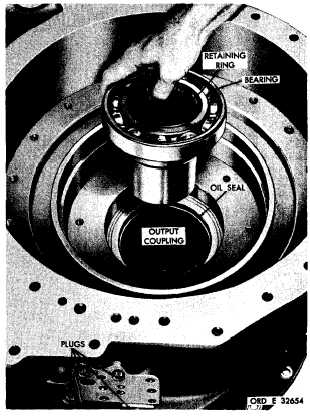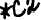Figure  234.  Removing  transmission  output  coupling and bearing
Custom Search*C2 C H A P    5,   SEC  X X V I I I T R A N S M I S S I O N     H O U S I N G     R E B U I LD P A R   2  0  3 - 2 0 6 Figure  234.  Removing  transmission  output  coupling and bearing .   P o s i t i o n    t h e    t r a n s m i s s i o n    h o u s i n g    to rest   on   its   bottom.   Using   a   hammer   and   soft drift,   drive   out   the   output   coupling   and   ball bearing   (fig.   234). Figure  235.  Transmission  output  coupling  and  bearing r e m o v ed .   R e m o v e    t h e    b e a r i n g    f r o m    t h e    o u t p ut coupling   (fig.   235). 2 0 5 .    I N S P E C T I O N    A N D    R E P A IR .   D o    n o t    r e m o v e    t h e    i n t e r n a l - r e t a i n i ng R e f e r    t o    p a r .    7 2    f o r    g e n e r a l    i n s p e c t i on ring   from   the   output   coupling   unless   replace- and    repair    recommendations.    Repair    and    re- m e n t    i s    n e c e s s a r y    ( f i g .    2 3 5 ) .    I f    n e c e s s a r y, b u i l d    p o i n t s    o f    m e a s u r e m e n t    f o r    f i t s ,    c l e a r- remove   the   retaining   ring. ances   and   wear   limits   are   indicated   by   small, lower   case   letters   in   fig.   375.   fold-out   4.   Re- .   Do   not   remove   the   oil   seal   from   the fer   to   par.   238   for   wear   limits   information. t r a n s m i s s i o n    h o u s i n g    u n l e s s    r e p l a c e m e n t    is n e c e s s a r y    ( f i g .    2 3 5 ) .    I f    n e c e s s a r y ,    d r i v e    t he 2 0 6 .    A S S E M B L Y    ( f i g .    3 7 5 ,    f o l d - o u t    4) oil   seal   out   of   the   housing. .   Install   any   plugs   removed   from   the .   I f    n e c e s s a r y ,    t h e    v a r i o u s    p l u g s    m ay transmission   main   housing   (figs.   233   and   235). b e    r e m o v e d    f r o m    t h e    h o u s i n g    t o    a i d    i n    t he c l e a n i n g    o f    t h e    o i l    p a s s a g e s    i n    t h e    h o u s i ng .   P o s i t i o n    t h e    t r a n s m i s s i o n    h o u s i n g    on (figs.   233   and   235). its   side.   Using   replacer   8351210,   install   the oil   seal,   lip   side   up,   in   its   transmission   hous- ing   bore   (fig.   235).   Be   sure   the   seal   is   firmly 2 0 4 .    C L E A N I NG seated   against   its   shoulder   in   the   housing. Refer   to   par.   71   for   cleaning   recommen- c .    I n s t a l l    t h e    b e a r i n g    o n t o    t h e    o u t p u t d a t i o n s. coupling   (fig.   235). 1 5 8Integrated Publishing, Inc. - A (SDVOSB) Service Disabled Veteran Owned Small Business